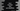# Dart HashSet skip and skipWhile methods explanation with example## Dart HashSet skip and skipWhile methods:

The skip and skipWhile methods are used to get iterable of a HashSet by skipping the first elements of a HashSet. This post will show you how to use the skip and skipWhile methods with examples.

### Dart HashSet skip method:

The skip method is used to get one iterable by skipping a given number of elements of a HashSet. The syntax of the skip method is:

``skip(int count) → Iterable<E>``

It takes one integer variable as its parameter. It will return one iterable by skipping the first count number of elements of the HashSet.

For example:

``````import 'dart:collection';

void main() {
final hashSet = HashSet.of([1, 2, 3, 4, 5, 6, 7, 8, 9]);

final newValuesIterable = hashSet.skip(4);

print('Original HashSet: \${hashSet}');
print('Iterable: \${newValuesIterable}');
}``````

If you run this program, it will print:

``````Original HashSet: {1, 2, 3, 4, 5, 6, 7, 8, 9}
Iterable: (5, 6, 7, 8, 9)``````

As you can see here, it created one iterable by skipping the first 4 numbers of the HashSet.

If the value of the variable count is equal or greater than the length of the HashSet, it will return one empty iterable.

For example,

``````import 'dart:collection';

void main() {
final hashSet = HashSet.of([1, 2, 3, 4, 5, 6, 7, 8, 9]);

final newValuesIterable = hashSet.skip(14);

print('Original HashSet: \${hashSet}');
print('Iterable: \${newValuesIterable}');
}``````

It will print:

``````Original HashSet: {1, 2, 3, 4, 5, 6, 7, 8, 9}
Iterable: ()``````

### Dart HashSet skipWhile method:

The skipWhile method of Dart uses a function to skip the first elements of a HashSet. The syntax of this method is:

``skipWhile(bool fn(E e)) → Iterable<E>``

It also returns one iterable. It uses the function fn to find out the starting elements to skip. It will skip all the elements for those the function returns true. Starting from the element for which it returns false, it will add all the elements of the HashSet to the iterable in the same order.

For example,

``````import 'dart:collection';

void main() {
final hashSet = HashSet.of([1, 2, 3, 4, 5, 6, 7, 8, 9]);

final newValuesIterable = hashSet.skipWhile((e) => e < 5);

print('Original HashSet: \${hashSet}');
print('Iterable: \${newValuesIterable}');
}``````

In this example, the skipWhile method will skip all the elements that are smaller than 5. So, the program will print:

``````Original HashSet: {1, 2, 3, 4, 5, 6, 7, 8, 9}
Iterable: (5, 6, 7, 8, 9)``````

If the function skips all the elements of the HashSet, it will return an empty iterable.

``````import 'dart:collection';

void main() {
final hashSet = HashSet.of(['a', 'b', 'c', 'd']);

final newValuesIterable = hashSet.skipWhile((e) => e.length > 1);

print('Original HashSet: \${hashSet}');
print('Iterable: \${newValuesIterable}');
}``````

Output:

``````Original HashSet: {c, a, b, d}
Iterable: (c, a, b, d)``````

As you can see here, the skipWhile function is skipping all the elements that have a length greater than one in the HashSet. But, since all the strings of the HashSet are of length one, it will skip all the elements and the iterable will hold all the strings of the HashSet.